JEE  >  VITEEE Maths Test - 4

# VITEEE Maths Test - 4

Test Description

## 40 Questions MCQ Test VITEEE: Subject Wise and Full Length MOCK Tests | VITEEE Maths Test - 4

VITEEE Maths Test - 4 for JEE 2023 is part of VITEEE: Subject Wise and Full Length MOCK Tests preparation. The VITEEE Maths Test - 4 questions and answers have been prepared according to the JEE exam syllabus.The VITEEE Maths Test - 4 MCQs are made for JEE 2023 Exam. Find important definitions, questions, notes, meanings, examples, exercises, MCQs and online tests for VITEEE Maths Test - 4 below.
Solutions of VITEEE Maths Test - 4 questions in English are available as part of our VITEEE: Subject Wise and Full Length MOCK Tests for JEE & VITEEE Maths Test - 4 solutions in Hindi for VITEEE: Subject Wise and Full Length MOCK Tests course. Download more important topics, notes, lectures and mock test series for JEE Exam by signing up for free. Attempt VITEEE Maths Test - 4 | 40 questions in 50 minutes | Mock test for JEE preparation | Free important questions MCQ to study VITEEE: Subject Wise and Full Length MOCK Tests for JEE Exam | Download free PDF with solutions
 1 Crore+ students have signed up on EduRev. Have you?
VITEEE Maths Test - 4 - Question 1

### A condition for a function y = f(x) to have inverse is that it should be

VITEEE Maths Test - 4 - Question 2

### The area bounded by the x-axis and the curve y = 4 x − x2 − 3 is

Detailed Solution for VITEEE Maths Test - 4 - Question 2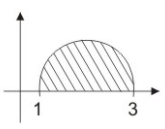y = 0, y = 4x - x2 - 3
⇒ 4x - x2 - 3 = 0
⇒ x2 - 4x + 3 = 0
⇒ (x - 1) (x - 3) = 0
⇒ x = 1, 3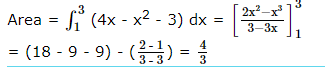VITEEE Maths Test - 4 - Question 3

### If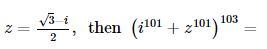VITEEE Maths Test - 4 - Question 4

If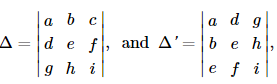then

VITEEE Maths Test - 4 - Question 5

If the function f is defined by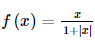then at what points is f differentiable

VITEEE Maths Test - 4 - Question 6

If z2 + z + 1 = 0, where z is a complex number then the value of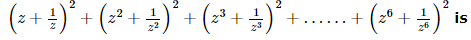VITEEE Maths Test - 4 - Question 7

If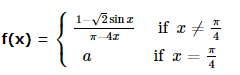is continuous at π/4 , then a =

VITEEE Maths Test - 4 - Question 8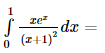Detailed Solution for VITEEE Maths Test - 4 - Question 8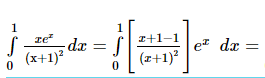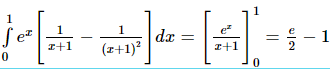VITEEE Maths Test - 4 - Question 9

The solution of the differential equation cos(x + y)dy = dx is

VITEEE Maths Test - 4 - Question 10

The solution of differential equation (dy/dx)=[(x(2logx+1))/(siny+ycosy)] is

VITEEE Maths Test - 4 - Question 11

The roots of the equation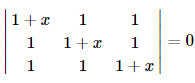are

Detailed Solution for VITEEE Maths Test - 4 - Question 11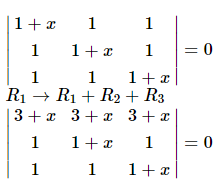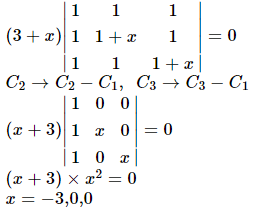VITEEE Maths Test - 4 - Question 12

The degree of the differential equation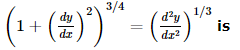Detailed Solution for VITEEE Maths Test - 4 - Question 12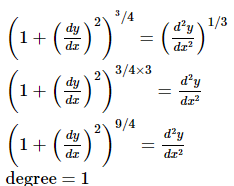VITEEE Maths Test - 4 - Question 13

The differential equation which represents the family of plane curves y=exp. (cx) is

Detailed Solution for VITEEE Maths Test - 4 - Question 13

y = ecx
dy dx = c. ecx
y' = cy

VITEEE Maths Test - 4 - Question 14

If x=a[(cost)+(log tan(t/2))], y=a sint, (dy/dx)=

VITEEE Maths Test - 4 - Question 15

The range of the function f(x) = x2 - x/x2 + 2x is

VITEEE Maths Test - 4 - Question 16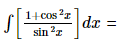Detailed Solution for VITEEE Maths Test - 4 - Question 16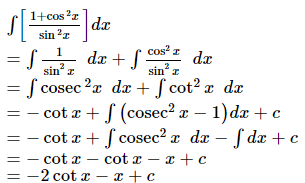VITEEE Maths Test - 4 - Question 17

If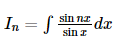where n > 1 , then In − In − 2

VITEEE Maths Test - 4 - Question 18

sec2(tan-1 2) + cosec2(cot-1 3) =

VITEEE Maths Test - 4 - Question 19

Let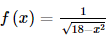What is the value of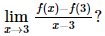VITEEE Maths Test - 4 - Question 20

The value of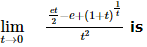Detailed Solution for VITEEE Maths Test - 4 - Question 20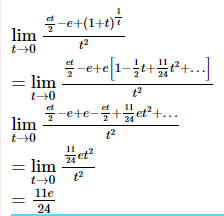VITEEE Maths Test - 4 - Question 21

Which is logically equivalent to "If today is Sunday, Matt cannot play hockey."?

Detailed Solution for VITEEE Maths Test - 4 - Question 21

Statements are logically equivalent to their contrapositives.

VITEEE Maths Test - 4 - Question 22

If A is a singular matrix, then A adj (A)

VITEEE Maths Test - 4 - Question 23

If A and B both are of order 2 x 3 , which one of these is not defined ?

VITEEE Maths Test - 4 - Question 24

Twelve students compete for a race. The number of ways in which first three prizes can be taken is

VITEEE Maths Test - 4 - Question 25

An unbaised die with faces marked 1,2,3,4,5 and 6 is rolled four times. Out of four face value obtained, the probability that the minimum face value is not less than 2 and the maximum face value is not greater than 5, is

VITEEE Maths Test - 4 - Question 26

A committee of 5 is to be chosen from a group of 9 people. What is the probability that a certain married couple serve together or not at all ?

VITEEE Maths Test - 4 - Question 27

Two dice are thrown simultaneously. The probability of obtaining a total score of seven is

Detailed Solution for VITEEE Maths Test - 4 - Question 27

There are six possible ways as to the number of points on the first die; and to each of these ways, there corresponding 6 possible numbers of points on second die.
Hence total number of ways S = 6 x 6 = 36
We now find out how many ways are favorable to the total of 7 points.
This may happen only in following ways:
(1, 6), (6, 1), (2, 5), (5, 2), (3, 4), (4, 3).
Hence, required Probability = 6/36 =1/6.

VITEEE Maths Test - 4 - Question 28

In a triangle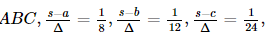, then b =

VITEEE Maths Test - 4 - Question 29

If the sum of n terms of the series 23 + 43 + 63 + ... ∞ is 3528, then n equals

VITEEE Maths Test - 4 - Question 30

The Quartile Deviation of the daily wages (in Rs) of 7 persons given below :
12, 7, 15, 10, 17, 19, 25 is

VITEEE Maths Test - 4 - Question 31

The S.D. of 5 scores
1 2 3 4 5 is

VITEEE Maths Test - 4 - Question 32

If x is positive, the greatest value of 5+4x-4x2 is

Detailed Solution for VITEEE Maths Test - 4 - Question 32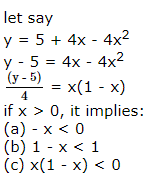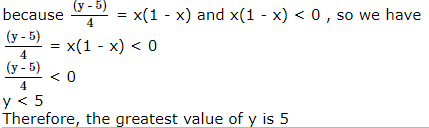VITEEE Maths Test - 4 - Question 33

The degree of the equation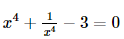is ____

Detailed Solution for VITEEE Maths Test - 4 - Question 33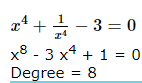VITEEE Maths Test - 4 - Question 34

A st. line which makes an angle of 600 with each of y and z axes, inclines with x-axis at an angle

VITEEE Maths Test - 4 - Question 35

The direction ratios of a normal to the plane through (1, 0, 0), (0, 1, 0), which makes an angle of π/4 with the plane x + y = 3 are

VITEEE Maths Test - 4 - Question 36

If sin2x+sin4x=2sin3x, then x=

VITEEE Maths Test - 4 - Question 37

Locus of centroid of the triangle whose vertices are (a cost, a sint), (b sint, -b cost) and (1, 0), where t is a parameter, is

VITEEE Maths Test - 4 - Question 38

In a ∆ABC, if A is the point (1, 2) and equations of the median through B and C are respectively x + y = 5 and x = 4, then B is

VITEEE Maths Test - 4 - Question 39

If θ is the angle between vectors a and b and |axb|=a.b, then θ=

VITEEE Maths Test - 4 - Question 40

The sine of the angle between the vectors 3i+2j-k and 12i+5j-5k is

## VITEEE: Subject Wise and Full Length MOCK Tests

1 videos|3 docs|73 tests
 Use Code STAYHOME200 and get INR 200 additional OFF Use Coupon Code
Information about VITEEE Maths Test - 4 Page
In this test you can find the Exam questions for VITEEE Maths Test - 4 solved & explained in the simplest way possible. Besides giving Questions and answers for VITEEE Maths Test - 4, EduRev gives you an ample number of Online tests for practice

## VITEEE: Subject Wise and Full Length MOCK Tests

1 videos|3 docs|73 tests